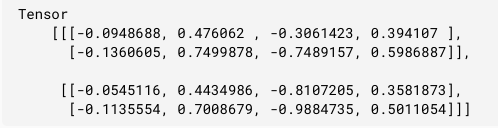Tensorflow.js tf.layers.gru() Function

• Last Updated : 17 Jun, 2021

Tensorflow.js is an open-source library developed by Google for running machine learning models and deep learning neural networks in the browser or node environment.

Tensorflow.js tf.layers.gru() function is used to create a RNN layer which consists of only one GRUCell and the apply method of this layer operates on a sequence of input tensors. The shape of input tensor must be atleast 2D and the first dimension must be time steps. gru is Gated Recurrent Unit.

Syntax:

tf.layers.gru(args)

Parameters:

• args: It specifies the given config object.
1. recurrentActivation: It specifies the activation function which will be used for the recurrent step. The default value of this parameter is hard sigmoid.
2. implementation: It specifies the implementation mode. It can be either 1 or 2. For superior performance implementation is recommended.

Return value: It returns a tf.layers.Layer

Example 1:

Javascript

 // Importing the tensorflow.js library const tf = require("@tensorflow/tfjs");   // Create a RNN model with gru Layerconst RNN = tf.layers.gru({units: 8, returnSequences: true});  // Create an input which will have 5 time stepsconst input = tf.input({shape: [5, 10]});const output = RNN.apply(input);  console.log(JSON.stringify(output.shape));

Output:

[null, 5, 8]

Example 2:

Javascript

 // Importing the tensorflow.js library const tf = require("@tensorflow/tfjs");   // Create a new model with gru Layerconst rnn = tf.layers.gru({units: 4, returnSequences: true});  // Create a 3d tensorconst x = tf.tensor3d([    [        [1, 2],        [3, 4],    ],    [        [5, 6],        [7, 8],    ],]);  // Apply gru layer to xconst output = rnn.apply(x);  // Print outputoutput.print()

Output:My Personal Notes arrow_drop_up